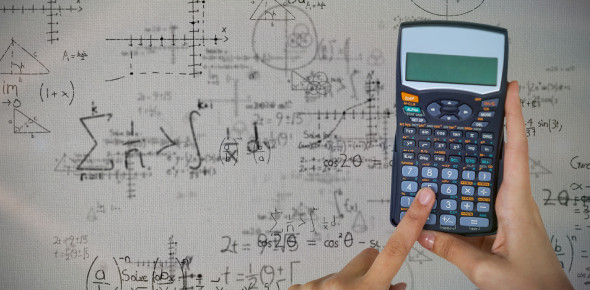# Quiz On Quadratic Equations For Class 10 Chapter 4

15 Questions | Attempts: 556
ShareSettingsHey, class 10th students, do you think your algebra is good? If yes, take up this quiz on quadratic equations created for class 10, consisting of Chapter 4 questions and answers. A quadratic equation can be arranged in a standard form ax^{2}+bx+c=0, where x represents an unknown number and a,b, and c represents known numbers. Here, you'll be asked questions based on factors and factorization. If you want to try this quiz and check how sharp your memory is, go ahead then. Play it now.

• 1.
The excluded value(s) for y2 + y + 5 / y + 4 is (are) ___.
• A.

Y = -4

• B.

Y = 4

• C.

Y = 0 ; y = -1

• D.

Y = 5 and y = 1

• 2.
The greatest common factor of x5y and x4 y2 is ___.
• A.

X5y2

• B.

X4y

• C.

Xy

• D.

X2y

• 3.
The factorization of 14a + 7b is ___
• A.

2(7a + 3b)

• B.

7a(2 + b)

• C.

7(2a + b)

• D.

14(a + b)

• 4.
Find the trinomial product of (4x + 3) (-2x - 5) : ___.
• A.

12x2 - 26x + 15

• B.

-8x2 + 14x - 15

• C.

-8x2 - 26x - 15

• D.

6x2 - 14x - 15

• 5.
Find the product of (4a + 3) (4a - 3) : ___.
• A.

12a2 - 9

• B.

8a2 + 9

• C.

16a2 - 9

• D.

16a2 + 2a - 9

• 6.
The binomial factors of 2x2 + 7x + 3 are ___.
• A.

(x + 3) (2x + 1)

• B.

(2x + 3) (x + 1)

• C.

(x + 3) (2x - 1)

• D.

(2x - 1) (x - 3)

• 7.
Factor 81n2 - 100 : ___.
• A.

(9n - 10)2

• B.

(81n + 10) (n - 10)

• C.

(9n - 10) (9n + 10)

• D.

(9n + 10)2

• 8.
The factors of 2 - 98n2 are ___.
• A.

-2(7n - 1) (7n + 1)

• B.

2(1 - 7n) (1 + 7n)

• C.

2(7n - 1)2

• D.

-2(49n2 - 1)

• 9.
The factors of 16y3 + 68y2 + 42y are ___.
• A.

2(4y + 7) (2y + 3)

• B.

(4y2 + 14y) (4y + 3)

• C.

4y(2y + 5) (2y + 2)

• D.

2y(2y + 7) (4y + 3)

• 10.
The formula for area is A = lw. If a rectangle has an area of 2x2 + x - 3, its dimensions are ___.
• A.

l: 2x - 1 w: x + 3

• B.

L: 2x - 3 w: x + 1

• C.

l: 2x + 1 w: x - 3

• D.

l: 2x + 3 w: x - 1

• 11.
A person purchased 5k + 2 items for a total cost of 35k2 + 29k + 6. The average cost per item was ___.
• A.

6k + 2

• B.

6k + 3

• C.

7k + 2

• D.

7k + 3

• 12.
• A.

A

• B.

B

• C.

C

• D.

D

• 13.
The indicated sum of y/3 + 5y/3 - 4y/3 is _________>
• A.

Y

• B.

2y/3

• C.

Y/3

• D.

10y/3

• 14.
The indicated quotient of ( - 1/3xy) ÷ (-3xy) is ____________.
• A.

1/3

• B.

1

• C.

- 1/9xy

• D.

1/ 9x2y2

• 15.
• A.

A

• B.

B

• C.

C

• D.

D

## Related TopicsBack to top
×

Wait!
Here's an interesting quiz for you.